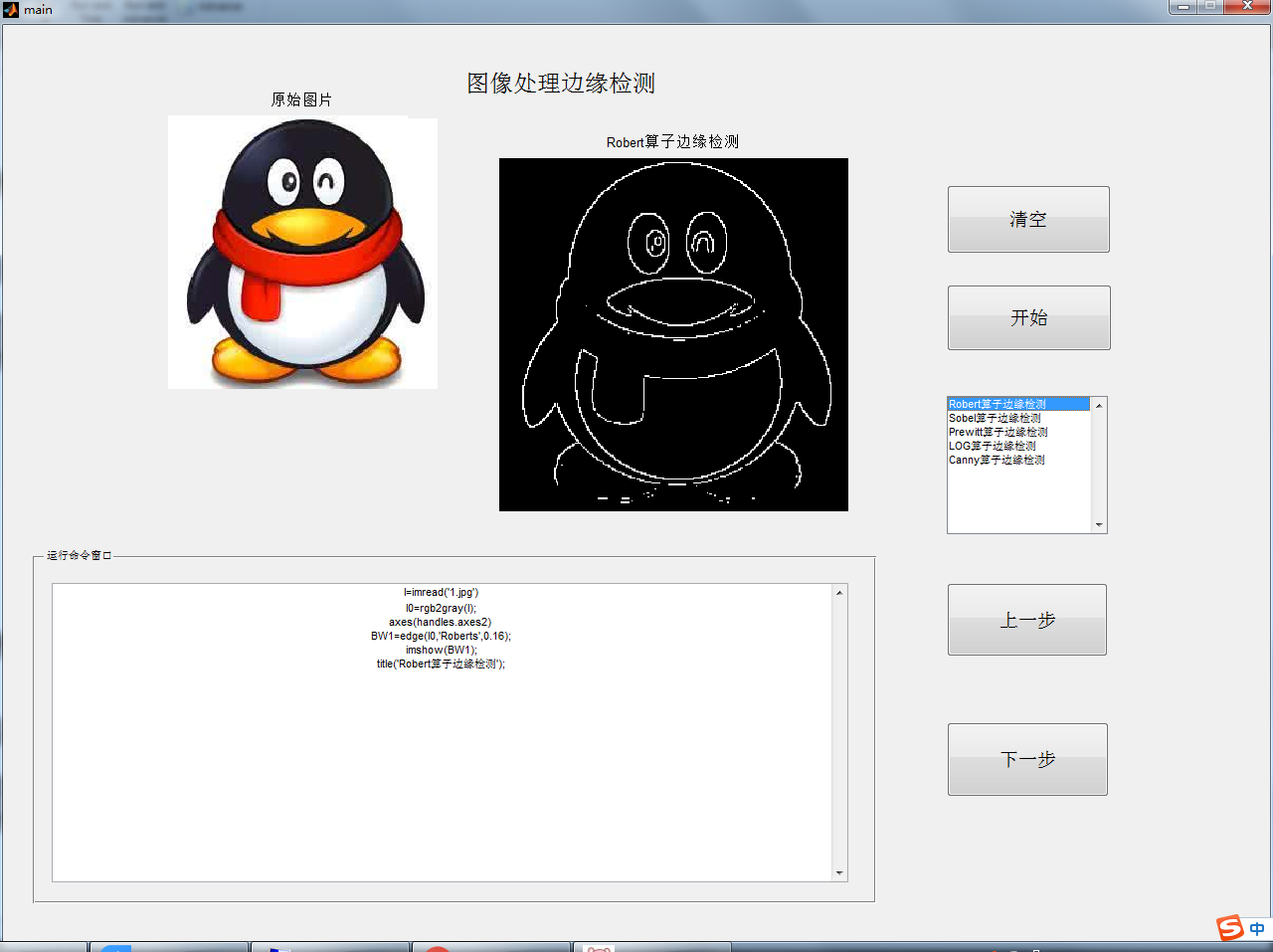# 【matlab GUI】GUI listbox的用法A= get(handles.listbox1,'value'); %A的值代表我们选的是第几个选项
switch A
case 1  % 当我们选的是1 时，给变量B赋个什么样的值，依次类推，最后B将是你想要的结果
I0=rgb2gray(I);
axes(handles.axes2)
BW1=edge(I0,'Roberts',0.16);
imshow(BW1);
title('Robert算子边缘检测')

str2 = ['I0=rgb2gray(I);', 10];
str3 = ['axes(handles.axes2) ', 10];
str4 = ['BW1=edge(I0,''Roberts'',0.16);',10];
str5=['imshow(BW1);',10];
str6=['title(''Robert算子边缘检测'');',10];
str = [str1, str2, str3, str4,str5,str6];
set(handles.edit1, 'string', str);

case 2
I0=rgb2gray(I);
axes(handles.axes2)
BW2=edge(I0,'Sobel',0.16);
imshow(BW2);
title('Sobel算子边缘检测')

str2 = ['I0=rgb2gray(I);', 10];
str3 = ['axes(handles.axes2) ', 10];
str4 = [' BW2=edge(I0,''Sobel'',0.16);',10];
str5=['imshow(BW2);',10];
str6=['title(''Sobel算子边缘检测'');',10];
str = [str1, str2, str3, str4,str5,str6];
set(handles.edit1, 'string', str);

case 3
I0=rgb2gray(I);
axes(handles.axes2)
BW3=edge(I0,'Prewitt',0.16);
imshow(BW3);
title('Prewitt算子边缘检测');

str2 = ['I0=rgb2gray(I);', 10];
str3 = ['axes(handles.axes2) ', 10];
str4 = ['BW3=edge(I0,''Prewitt'',0.16);',10];
str5=['imshow(BW3);',10];
str6=['title(''Prewitt算子边缘检测'');',10];
str = [str1, str2, str3, str4,str5,str6];
set(handles.edit1, 'string', str);
case 4
I0=rgb2gray(I);
axes(handles.axes2)
BW4=edge(I0,'LOG',0.012);
imshow(BW4);
title('LOG算子边缘检测')

str2 = ['I0=rgb2gray(I);', 10];
str3 = ['axes(handles.axes2) ', 10];
str4 = ['BW4=edge(I0,''LOG'',0.012);',10];
str5=['imshow(BW4);',10];
str6=['title(''LOG算子边缘检测'');',10];
str = [str1, str2, str3, str4,str5,str6];
set(handles.edit1, 'string', str);
case 5
I0=rgb2gray(I);
axes(handles.axes2)
BW5=edge(I0,'Canny',0.2);
imshow(BW5);
title('Canny算子边缘检测')

str2 = ['I0=rgb2gray(I);', 10];
str3 = ['axes(handles.axes2) ', 10];
str4 = ['edge(I0,''Canny'',0.2);',10];
str5=['imshow(BW5);',10];
str6=['title(''Canny算子边缘检测'');',10];
str = [str1, str2, str3, str4,str5,str6];
set(handles.edit1, 'string', str);
end

09-0103-06
12-08
04-151万+
02-241万+
11-182412
01-011856
12-19441
12-19274
10-101万+
09-293207
06-097万+
11-281万+
12-271961
03-02388
02-06239